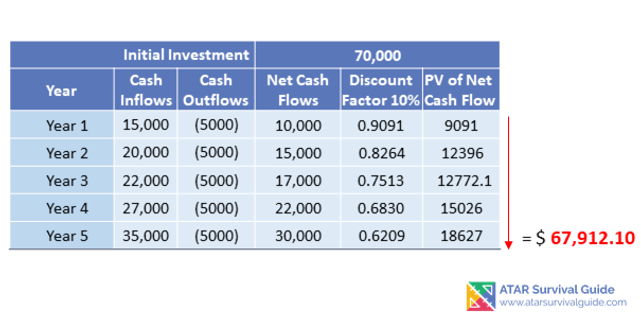### Capital Expenditure

#### NPV with Differing Cash Flows

###### Content ContributorsPriya KaurChristian Bien

# Learning Objectives###### ​###### How Do We Calculate Net Present Value Where the Cash Flows are Different Each Year?The previous page show how to calculate NPV where the Net Cash Flow is consistent each year.

For an asset where cash flows are different each year, the NPV is calculated by applying the discount factor individually to the net cash flow of each period.###### Worked Example: NPV Calculation with differing Net Cash FlowsBelow is an example of how to calculate the NPV where cash flows are not consistent each year. Christian Inc. is approached by an overseas company to invest in a project lasting 5 years. Christian Inc will have to contribute \$70,000 upfront.

This project is estimated to provide the following economic benefits and outflows in each year of the project's life as seen in the table. The expected Cost of Capital is 10%. Calculate the NPV of this project and determine if Christian Inc. should go ahead with this project.###### Step 1: Calculate the Net Cash FlowsThe first step is to find the Net Cash Flows of each year by Subtracting the Cash Outflows from the Cash Inflows. E.g.

In Year 1: \$15,000 (Inflows) - \$5,000 (Outflows)= \$10,000 (Net Cash Flow).

In Year 2: \$20,000 - \$5000 = \$15,000 and so on.###### Step 2: Applying the Discount FactorSince the Net Cash Flows are different each year, they will need to be discounted individually using their respective rates rates to give their Present Value.

To do this, the Net Cash Flow for each Year is multiplied with the figure that corresponds with the Cost of Capital (Discount Factor) and the Period. Net Cash Flow for the period × Discount Figure = Present Value of Future Cash Flow We know that the Cost of Capital (Discount Factor) is 10%.

For Year 1: 10,000 (Net Cash Flow) is multiplied by 0.9091 (Discount Figure)- as this figure corresponds with the Discount Factor of 10% for Period 1, resulting in = 9091 as the Present Value of the Future Cash Flow for this Year (Refer to the image at the bottom of the page)

For Year 2: 15,000 (Net Cash Flow) × 0.8264 (Discount Figure corresponding with Discount Factor of 10% for Period 2) = 12,396 (Present Value of the Future Cash Flow for this Year. * For different Cash Flows each year, the discount figures should be attained from the 'Present Value of \$1 at the end of future periods' table provided in the Accounting and Finance ATAR Specifications Booklet.###### Step 3: Calculate the Total Present Value of the Future Cash FlowsOnce the Present Value for the Net Cash Flows of each Year is calculated, add them all together to find the Total Present Value of Future Cash Flows throughout the life of the Project. 9091 ( Y1) + 12396 (Y2) + 12772.1 (Y3) + 15026 (Y4) + 18627 (Y5) = \$ 67,912.10 (Total PV of Future Cash Flows).###### Step 4: Finding the Net Present Value of the ProjectAs mentioned before, the formula for the Net Present Value = (PV of Future Cash Flows) - (PV Cost of Capital Investment). In Step 3, we have calculated the Present Value of Future Cash Flows to be = \$67,912.10

From the question we are told the cost of the project contribution is \$70,000. By substituting these figures in the NPV Formula: = \$67,912.10 (PV of Future Cash Flows) - \$70,000 (PV Cost of Capital Investment) = - \$2087.90 ----> This is the Net Present Value.###### Step 5: Interpretation of the Net Present ValueThe final NPV is a negative figure of - \$2087.90.

This can be interpreted as the project will make \$2087.90 less than the minimum 10% return expected from the Cost of Capital, therefore this Investment should be Rejected.###### Reference: Discount Factor Table in the Case of Differing Net Cash FlowsThis image of the table has been extracted from the Specifications booklet. We know that the Cost of Capital (Discount Factor) is 10%.

For Year 1: 10,000 (Net Cash Flow) is multiplied by 0.9091 (Discount Figure)- as this figure corresponds with the Discount Factor of 10% for Period 1, resulting in = 9091 as the Present Value of the Future Cash Flow for this Year.